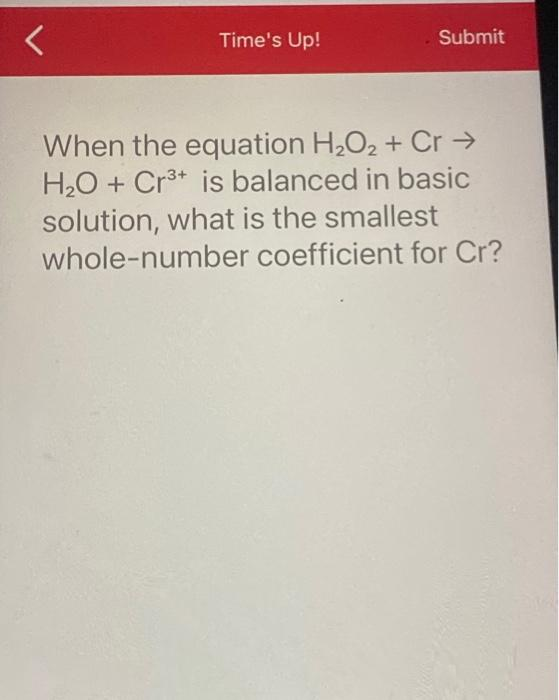Home / Expert Answers / Chemistry / time-39-s-up-submit-when-the-equation-ho-cr-ho-cr-is-balanced-in-basic-solution-w-pa535

# (Solved): Time's Up! Submit When the equation HO + Cr HO + Cr+ is balanced in basic solution, w ...

Time's Up! Submit When the equation H₂O₂ + Cr → H₂O + Cr³+ is balanced in basic solution, what is the smallest whole-number coefficient for Cr?When the equation is balanced in basic solution, what is the smallest whole-number coefficient for ?

We have an Answer from Expert

In this step we will balance the equation

Step 1:

Break the equation into oxidation and reductio half reaction.

Reduction :

Oxidation :

Step 2:

Now balance the element other than H and O

Reduction :

Oxidation :

Step 3: